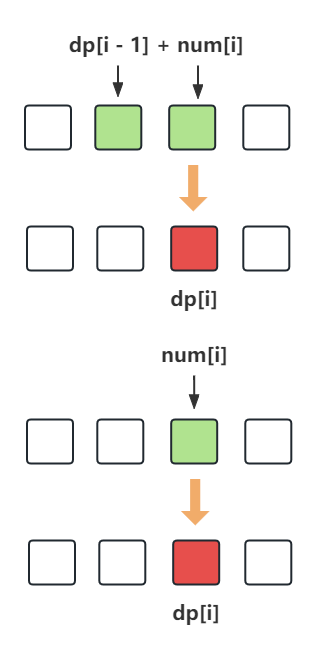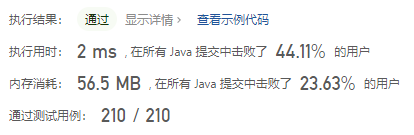#### 53. 最大子数组和

###### 来源: 每日一题 2023.07.20 延伸

``````输入：nums = [-2,1,-3,4,-1,2,1,-5,4]

``````

``````输入：nums = 

``````

``````输入：nums = [5,4,-1,7,8]

```

``````

1 <= nums.length <= 105
-104 <= nums[i] <= 104

``````
```Java
class Solution {
public int maxSubArray(int[] nums) {

}
}
``````

#### 分析与题解

• 动态规划

这个题目算是动态规划入门级别的问题了, 整体来说比较简单, 我们使用 dp[i] 代表当前 第 i 个元素的子数组最大值, 那么 dp[i] 只有两种情况

• 只取 num[i] (最少要有一个元素)

• num[i] + dp[i -1]

• 两者取最大即可.代码如下所示.

``````class Solution {
public int maxSubArray(int[] nums) {
int length = nums.length;
int[] dp = new int[length];
dp = nums;
int result = nums;
for(int i = 1; i < length; i++) {
dp[i] = Math.max(nums[i], nums[i] + dp[i-1]);
result = Math.max(dp[i], result);
}
return result;
}
}
``````

复杂度分析:

• 时间复杂度: O(n)
• 空间复杂度: O(n)

结果如下所示.IT界无底坑洞栋主 欢迎加Q骚扰:676758285